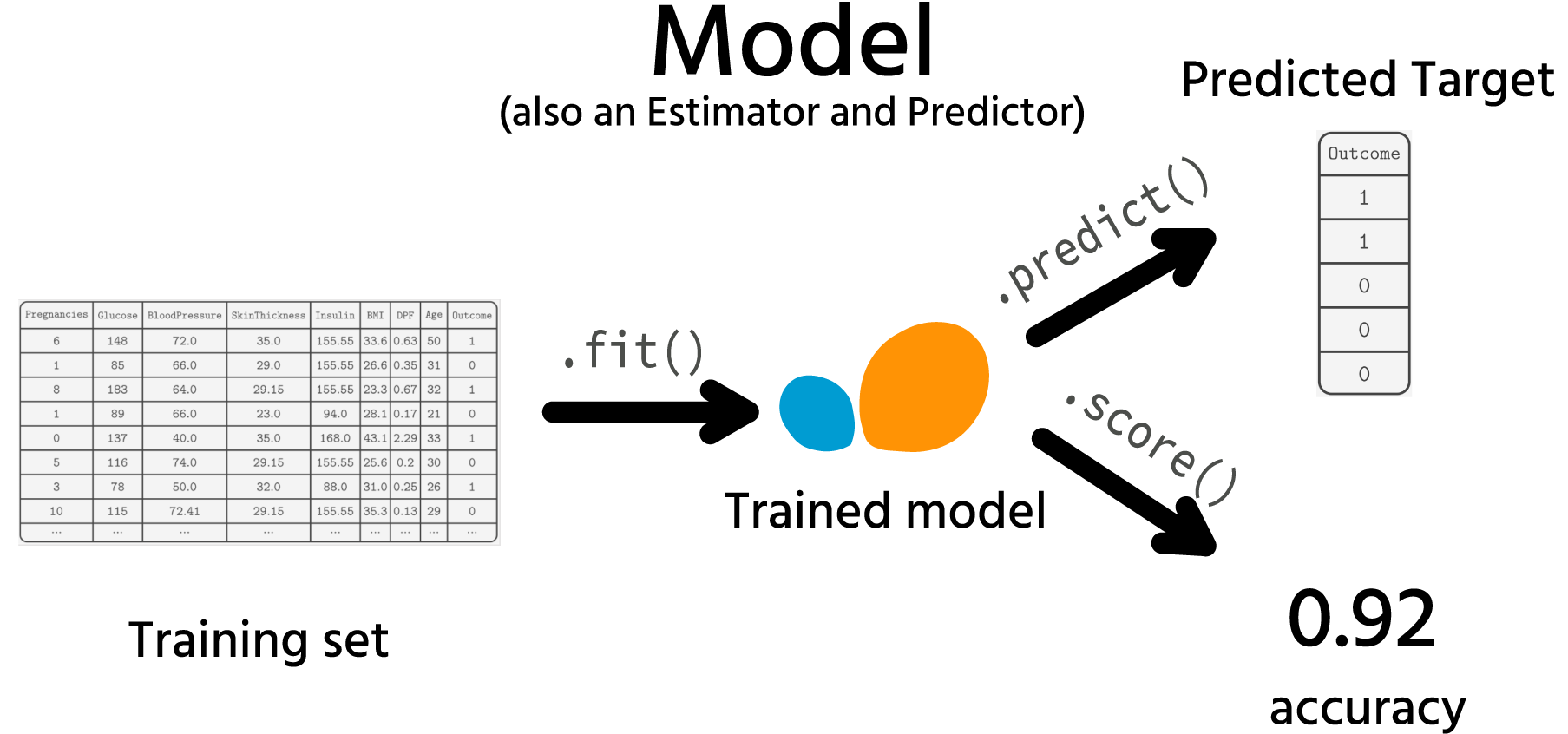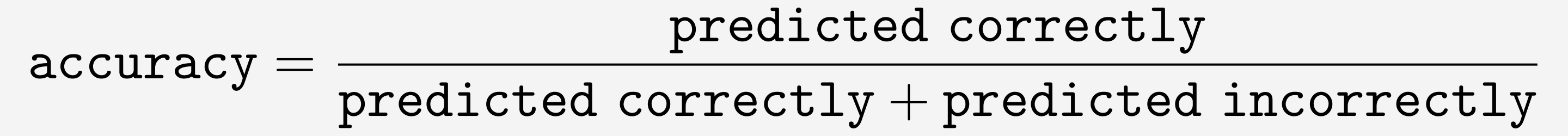Course Content

ML Introduction with scikit-learn

##Models

You already know the basics of preprocessing your data and how to build pipelines. That's great! Now we can move to the fun part, Modeling!

Let's recap what a model is. In Scikit-learn, it is an Estimator that has both `.predict()` and `.score()` methods (and since it is an Estimator, the `.fit()` method is also present).## .fit()

Once the data is preprocessed and ready to go to the model, the first step of building a model is training a model.
This is done using the `.fit(X, y)`.

Note

To train a model performing a supervised learning task (e.g., regression, classification), you need to pass both `X` and `y` to the `.fit()` method.
If you are dealing with an unsupervised learning task (e.g., clustering), it does not require labeled data, so you can only pass the `X` variable, `.fit(X)`. However, using `.fit(X, y)` will not raise an error. The model will just ignore the `y` variable.

During the training, a model learns everything it needs to perform predictions.
What a model learns and how long it will train depends on the algorithm you choose. For each task, there are many models based on different algorithms. Some of them train slower, some – faster.
But overall, training is usually the most time-consuming thing in Machine Learning, and if the training set is large, a model can train for minutes, hours, or even days.

## .predict()

Once the model is trained using the `.fit()` method, it can perform predictions.
Predicting is as easy as calling the `.predict()` method:

Usually, you want to predict a target for new instances, `X_new`.

## .score()

The `.score(X, y)` method is used to measure a trained model's performance. Usually, it is calculated on the test set (the following chapters will explain what it is). Here is the syntax:

The `.score()` method requires actual target values (`y_test` in the example). It calculates the prediction for `X_test` instances and compares this prediction with the true target (`y_test`) using some metric.
By default, this metric is accuracy for classification.The next chapter will briefly explain how the `KNeighborsClassifier` model works and what it does during training and predicting.

Everything was clear?

Section 4. Chapter 1